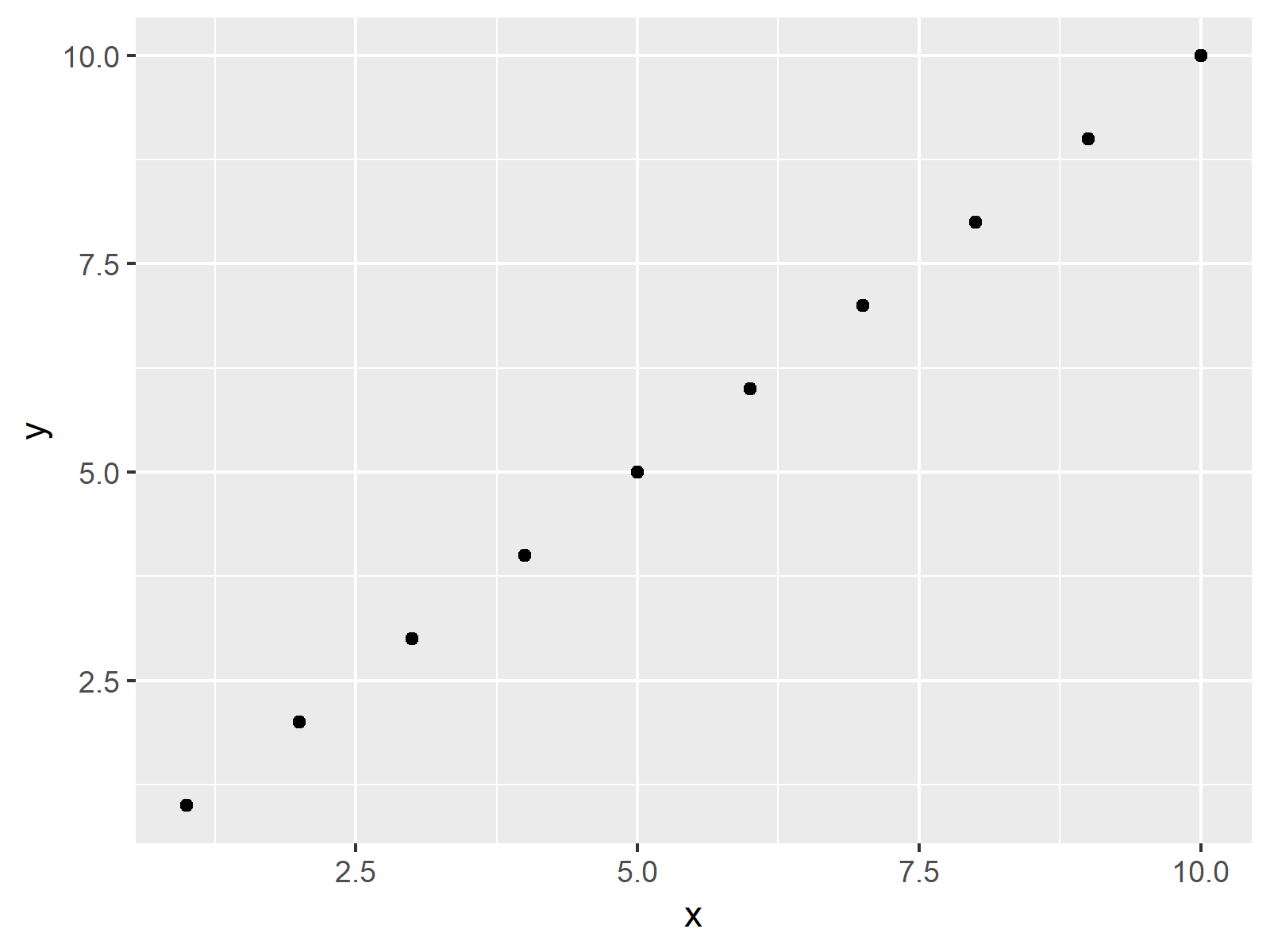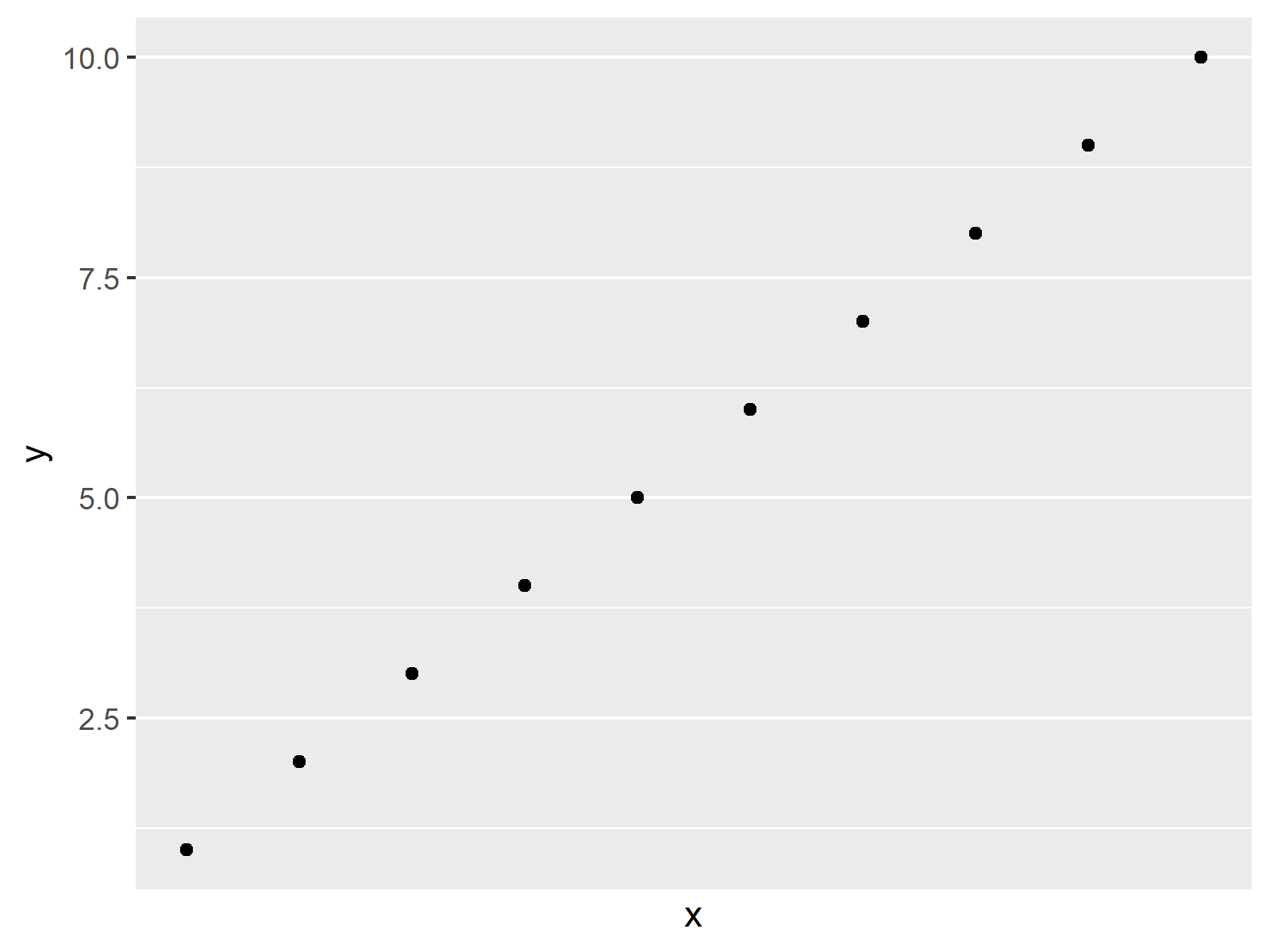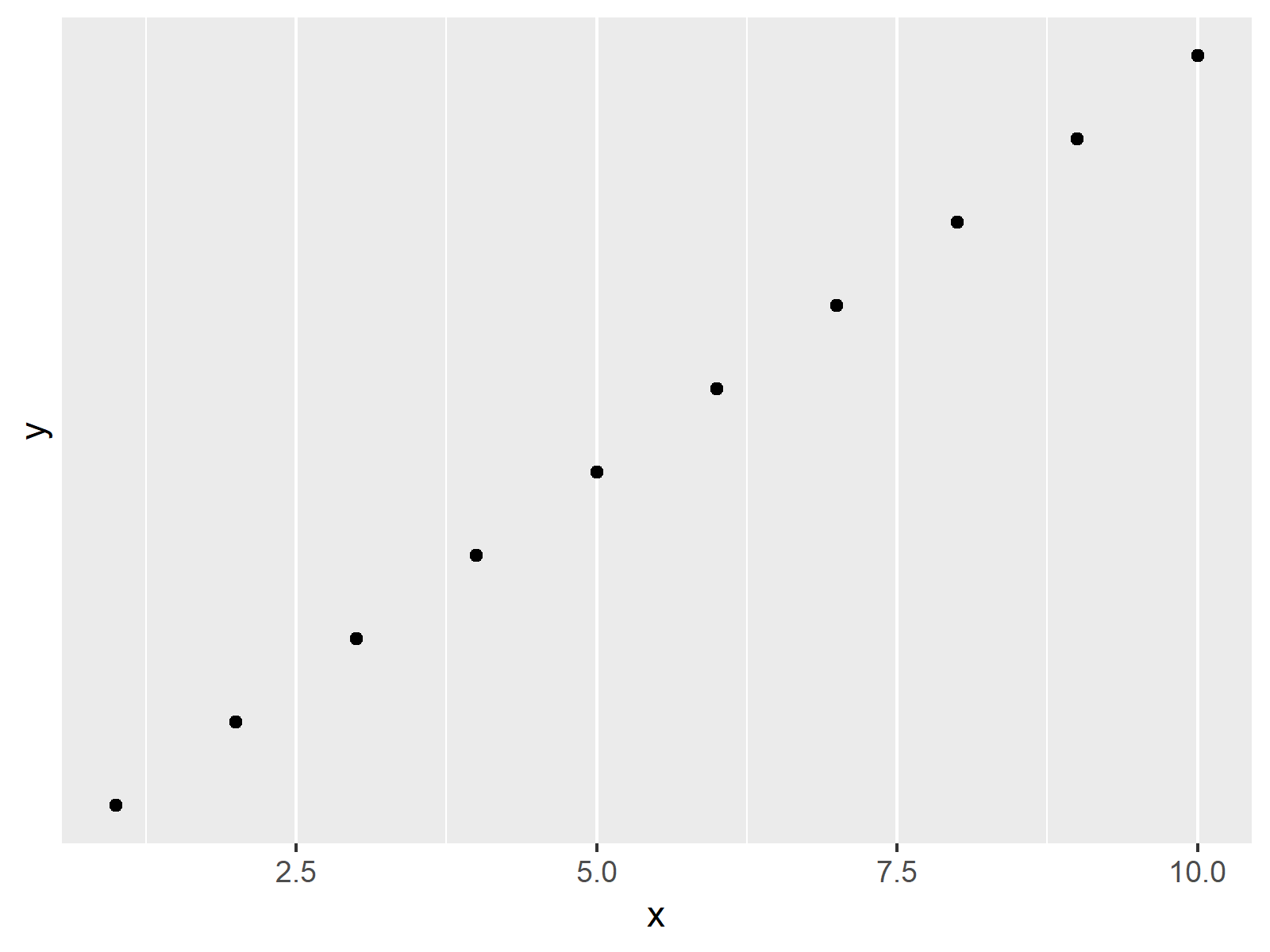# Remove Vertical or Horizontal Gridlines in ggplot2 Plot in R (2 Examples)

In this R tutorial you’ll learn how to delete lines in a ggplot2 background grid.

The page will consist of these topics:

Let’s dig in!

## Example Data, Packages & Basic Graph

The following data will be used as basement for this R programming tutorial:

```data <- data.frame(x = 1:10,               # Example data
y = 1:10)
data                                       # Show example data in console
#     x  y
# 1   1  1
# 2   2  2
# 3   3  3
# 4   4  4
# 5   5  5
# 6   6  6
# 7   7  7
# 8   8  8
# 9   9  9
# 10 10 10```

Have a look at the previous output of the RStudio console. It shows that the example data consists of two numeric rows both ranging from the values 1 to 10.

We also have to install and load the ggplot2 package, if we want to apply the corresponding functions:

```install.packages("ggplot2")                # Install & load ggplot2
library("ggplot2")```

Now, we can draw our data as follows:

```ggp <- ggplot(data, aes(x, y)) +           # Basic ggplot2 plot with gridlines
geom_point()
ggp                                        # Draw plot```As shown in Figure 1, we created a ggplot2 plot with default grid background with the previous R syntax.

## Example 1: Create ggplot2 Plot without Vertical Lines

In Example 1, I’ll explain how to remove the vertical lines in a ggplot2 background grid using the scale_x_continuous function.

`ggp + scale_x_continuous(breaks = NULL)    # ggplot2 plot without vertical lines`Figure 2 shows the output of the previous code – A ggplot2 graphic without vertical background gridlines.

## Example 2: Create ggplot2 Plot without Horizontal Lines

In this Section, I’ll illustrate how to suppress the horizontal gridlines of a ggplot2 plot. In this example, we are using the scale_y_continuous function. Besides that, the R syntax is the same as in Example 1:

`ggp + scale_y_continuous(breaks = NULL)    # ggplot2 plot without horizontal lines`As shown in Figure 3, we created another ggplot2 plot, but this time without the lines from the left to the right side of the plot.

## Video & Further Resources

In case you need more info on the content of this tutorial, you may have a look at the following video of my YouTube channel. I’m explaining the examples of this article in the video.

Please accept YouTube cookies to play this video. By accepting you will be accessing content from YouTube, a service provided by an external third party.If you accept this notice, your choice will be saved and the page will refresh.

In this R tutorial you learned how to suppress ggplot2 gridlines. In case you have any further comments and/or questions, please tell me about it in the comments section.

Subscribe to the Statistics Globe Newsletter